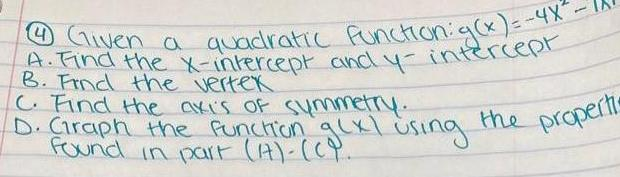Question:

# 4 Given a quadratic function g x 4x A Find the x intercept

Last updated: 3/18/20234 Given a quadratic function g x 4x A Find the x intercept and y intercept B Find the vertex C Find the axis of symmetry D Graph the function gcxl Found in part 1 CY using the properti